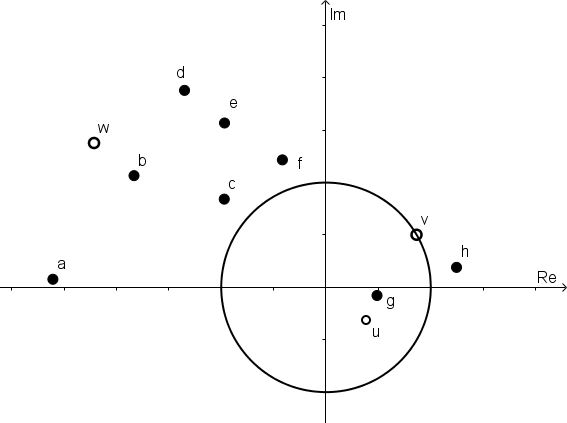# Complex Puzzle

##### Age 14 to 18Challenge Level

This resource is part of our Adventures with Complex Numbers collection

This activity follows on from Into the Wilderness.In this diagram, there are several points and the unit circle (the circle with centre at the origin and radius 1).

The points $a$ to $h$ are the sum or product of combinations of $u$, $v$ and $w$.
Can you work out which of the expression below describes each point?

$u + v$
$u + w$
$v + w$
$u + v + w$
$uv$
$uw$
$vw$
$uvw$

You might now like to start Mapping the Territory.

This problem was inspired by an exercise in Complex Analysis by Ian Stewart and David Tall.